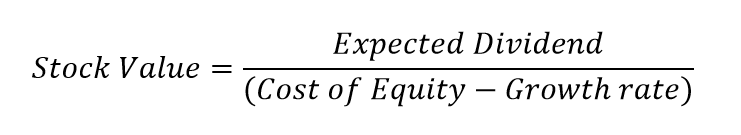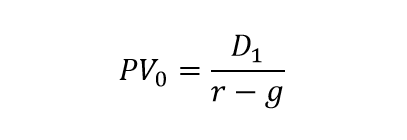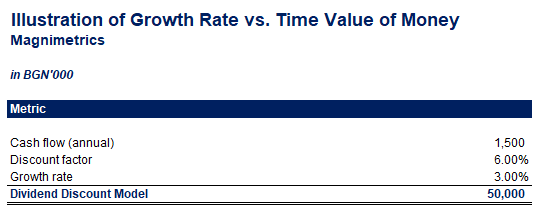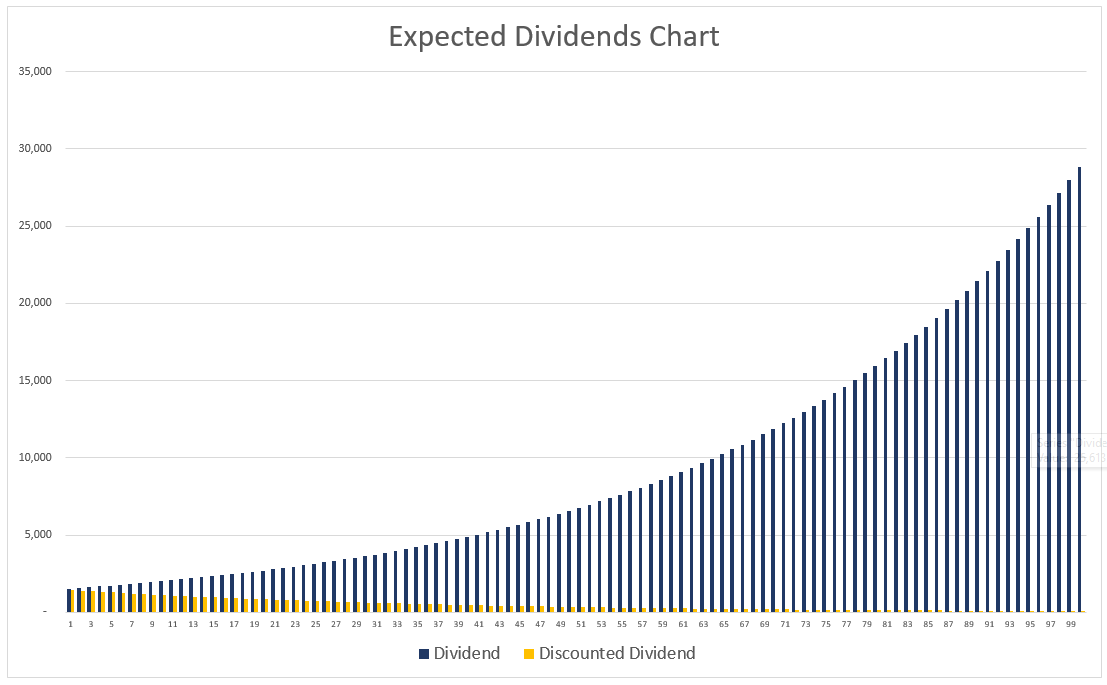## Introduction to the Dividend Discount Model

The Dividend Discount Model (DDM) is used to estimate the price of a company’s stocks. The model is based on the theory that the present value of the stock is equal to the present value of all future dividend payments when discounted back to the present.

If the present value calculated under the DDM is higher than the current trading price, the stock is undervalued and qualifies for a ‘buy’ decision.

## The Dividend Discount Model (DDM)

Companies generally provide services or produce goods, to make profits. The business usually uses these profits to distribute dividends to the shareholders.

The Discount Dividend Model stipulates that the value of the company is the present value of all dividends it will ever pay to the shareholders. The method uses the principle of the time value of money – if we can get 100 euros now and in one year, we will prefer to receive it now, as we will have one year to generate a return on this amount.Where:

• FV is the future value of the cash flow;
• PV is the present value of the cash flow;
• r is the rate of return we can achieve on investment.

Rearranging the formula, we can calculate the present value from the future value:The Dividend Discount Model for valuation uses the time value of money concept to calculate the Net Present Value (NPV) of the cash flows a company will generate in the future in the form of dividends to shareholders.

### Estimating Dividends

Trying to forecast future dividend payouts can be a cumbersome task. It involves making assumptions or trying to identify and calculate trends based on past dividend payments.

Analysts usually assume the company will have a fixed growth rate and treat it as perpetuity (a regular stream of equal cash flows with no end). We typically calculate the growth rate based on historical data.

### Discount factor

Shareholders bear the risk of a decline in the value of their purchased stock in companies. Therefore they expect a return on their investment (compensation). A company’s cost of equity represents the expected remuneration investors demand in exchange for bearing the risk of ownership.

Analysts can estimate the discount factor via the Capital Asset Pricing Model (CAPM) or the Dividend Growth Model. We consider the rate of return less the expected annual growth to be the effective discounting factor for a company’s dividends.

DDM shows us how much the stock should cost if we expect it to generate our desired return.

### Growth Rate

We can calculate the growth rate as Return on Equity multiplied by the retention rate (the opposite of the dividend payout ratio). The dividend is paid out of the profit of the company, so it cannot exceed it.

This means that the rate of return of the stock has to be larger than the expected dividend growth rate for future cash flows. Otherwise, we will get an unsustainable model with negative stock prices, which is not possible in real-life scenarios.

We can easily illustrate how growth increases the nominal value of the dividend payout while the time value of money concept decreases its purchasing power.## Dividend Discount Model Formulae

The model has many variants with different complexity. We will take a look at the most commonly used ones below.

### Gordon Growth Model

This variation wears the name of Myron J. Gordon, who proposed it, and it is the most common version of the Dividend Discount Model.

To calculate it we use the following formula:The Gordon Growth variation assumes a stable dividend growth rate for future periods. Presenting the above method in a more concise mathematical way, we have:Where:

• PV0 is the present value of the stock;
• D1 is the dividend at the end of the first period;
• r is the discount rate;
• g is the expected growth rate.

### No-growth Dividend Discount Model

This variant expects the dividend to stay the same, with no growth. It is the same as the present value of perpetuity:Where:

• PV0 is the present value of the stock;
• D1 is the dividend at the end of the first period;
• r is the discount rate.

The present value of the stock here can only change if the required rate of return changes.

### Variable Growth Rate DDM

We also refer to this variation as a Multi-Stage Dividend Discount Model. It is the closest one to reality, as it expected different growth phases for the company. Most commonly, analysts assume three stages:

• Initial high growth rate when the company is new and attractive to investors;
• Transition to slower growth as the company approaches maturity;

We can calculate each phase as a separate constant-growth DDM, and then add them together. We need to remember that we have to discount these future stages to their present value.

### One-period Dividend Model

This one is less popular, and analysts employ it to estimate the intrinsic value of stocks to be sold in one year. We calculate it via the following formula:Where:

• PV0 is the present value of the stock;
• D1 is the dividend at the end of one year;
• SP1 is the selling price of the stock at the end of one year;
• r is the discount rate.

### Multi-period Dividend Model

This version is an extension of the One-period Dividend Model. The investor expects to hold the stock for multiple periods. We calculate it using the following formula:Where:

• PV0 is the present value of the stock;
• D1 to Dn are the dividends at the end of each period;
• SPn is the selling price of the stock at the end of the nth period;
• r is the discount rate.

The main challenge of the Multi-period Dividend Model is forecasting the dividend payments for the multiple future periods, as well as the selling price of the stock at the end.

## Issues With The Dividend Discount Model

While analysts and investors commonly use the method, it is essential to remember that it has some disadvantages:

• The most popular variant assumes a constant growth rate each year, which is highly unlikely;
• The model is susceptible to input changes, since the difference between the discount factor and the growth rate is very small, making tiny changes highly impactful;
• Applying the technique to small-dividend stocks tend to yield inaccurate results;
• The Dividend Discount Model is mostly suitable for mature, stable companies, and it is not intended for start-ups and companies in the high-growth stage of development;
• The model may not be indicative of the company’s earnings, as businesses aim for consistent payouts and can sometimes even use borrowed funds to distribute dividends;
• It has the underlying assumption that we can use dividends as an accurate measure of the cash flows returned to shareholders.

Even though the model has some limitations, it has advantages as well:

• The method has a strong theoretical background and a sound mathematical model;
• The Dividend Discount Model eliminates potential subjectivity;
• Dividends can influence the stock price. Therefore companies tend to keep them in line with the fundamental metrics of the company, meaning our inputs are less susceptible to being tampered with;
• Analysts believe that the Dividend Discount Model is most applicable to companies that pay dividends; however, we can still apply it to firms that do not distribute profits to their shareholders by making assumptions about what dividend these companies can pay.

## Example

We can review the following case to get a better grasp of the concept behind the Dividend Discount Model. We have the opportunity to buy stock in a mature company that pays out dividends regularly, on an annual basis. At the end of next year, the expected dividend is 7,500 euros. We forecast a growth rate of 3.50% based on historical data. We also have a required rate of return of 8.50%, which is the hurdle rate we use for our investment portfolio.

As the company has existed for over 150 years, we expect it to continue to operate and distribute dividends for an indefinite future. Therefore we can apply the Gordon Growth Model as the most common variant of the Dividend Discount Model.The fair value of the stock price is 150 thousand euros. Please, be aware that this is the fair value that would satisfy our specific hurdle rate.

If the asking price for the stock is lower than this fair value, this can be one indication that it is a viable investment opportunity.

Let’s take a look at a different scenario, where we can apply the Multi-period Dividend Discount Model. This time we can invest in a stock that will pay out various dividends for five years, and we plan to sell it at the end of the 5th year. After performing some forecasts on the earning of the company for the next five years, we estimate the following dividend payments for each period, as well as selling price after the last period at the amount of 45 thousand euros. Applying the Multi-period DDM and our required rate of return of 8.50%, we arrive at a fair stock price of 52,566 euros.Since we have the option to purchase this stock for 55 thousand euro, it seems that we should not proceed with the investment.

### Growth Rate and Time Value of Money

We can easily illustrate the effect of the dividend growth rate and the time value of money concept. If we apply the DDM model for a regular cash flow of 1,500 euros, an expected growth rate of 3.00%, and a discount factor of 6.00%, we get a present value of 50 thousand euros.Let us assume we are not aware of the Dividend Discount Model. What we can alternatively do is calculate the dividends for a large number of periods, applying the growth rate. Then we can discount all the cash flows and arrive at the present value for the entire stock investment.

We calculate the DCF for the next 100 years, and already the discounted present value of 48,583 euros is getting close to the result of our DDM calculation above.The table for 100 periods and the following graphic illustrate the effect of the growth rate and the time value of money concept.

As we move into the future, the dividend payout grows, giving us almost 950 thousand euros of profit distributions in a century. However, the discounted present value dramatically diminishes as we move further in time, due to the time value of money concept, essentially becoming close to zero.As we cannot calculate the dividends and discounted cash flows for an infinite number of years, we employ the Dividend Discount Model instead.

You can look at both examples in the Excel files attached at the end of the article.

## Conclusion

All variants of the Dividend Discount Model allow us to value shares outside of the current market conditions. This allows us to compare even companies from very different industries.

We should remember that the model is only one of a vast plethora of valuation tools that are available to us. It can indicate undervalued stocks and an underlying investment opportunity, but we should always use it in corroboration with other techniques and information. Other methods that we can combine with DDM include Return on Equity (ROE), Price-to-earnings ratio, Dividend payout ratio, and others.

The DDM is developed under the assumption that the intrinsic value of a stock reflects the present value of all future cash flows. It makes it easy to calculate a fair stock price, at least from a mathematical point of view, requiring minimum input variables. However, it relies on assumptions that are hard to forecast.

Generally, an analyst needs to forecast future dividend payments, cost of equity capital, and future dividend growth rate. Doing so in real life is close to impossible, and we need to be fully aware that the theoretical stock price from applying the method can be far from reality.

## Get a FREE Excel Benchmark Analysis Template## FCCA, FMVA

Hi! I am a finance professional with 10+ years of experience in audit, controlling, reporting, financial analysis and modeling. I am excited to delve deep into specifics of various industries, where I can identify the best solutions for clients I work with.

In my spare time, I am into skiing, hiking and running. I am also active on Instagram and YouTube, where I try different ways to express my creative side.

The information and views set out in this publication are those of the author(s) and do not necessarily reflect the official opinion of Magnimetrics. Neither Magnimetrics nor any person acting on their behalf may be held responsible for the use which may be made of the information contained herein. The information in this article is for educational purposes only and should not be treated as professional advice. Magnimetrics and the author of this publication accept no responsibility for any damages or losses sustained in the result of using the information presented in the publication.